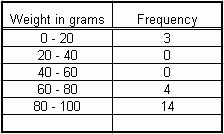Email us to get an instant 20% discount on highly effective K-12 Math & English kwizNET Programs!

#### Online Quiz (WorksheetABCD)

Questions Per Quiz = 2 4 6 8 10

### Middle/High School Algebra, Geometry, and Statistics (AGS)9.1 Introduction to Statistics

 Statistics is the science which is concerned with the collection, presentation and drawing of conclusions from numerical data. The numerical facts and observations collected are done with a specific purpose. Scope of Statistics i) Statistics are a sum total of observations. A single observation cannot form a statistic. ii) Statistics are expressed quantitatively and not qualitatively. Limitations of Statistics i) Statistics laws are not exact. Actually they are true on averages only. ii) Data collected for some specific purpose may not be suited for another purpose. Presentation of data i)Data obtained in the original form is called Raw or ungrouped data ii)Data arranged in an ascending or descending fashion is called Arranged data Variate The quantity that may vary from observation to observation is called a variate Classes When we divide the values of the variate into convenient groups, we call them Classes Frequency The number of times the variate occurs is called the frequency of that variate Frequency Table A table showing the groups and their corresponding frequencies is called the frequency table Let us take an example of the frequency table with continuous intervalsClass intervals In this example there are 5 class intervals Ist class interval = 0-20 IInd class interval = 20-40 IIIrd class interval = 40-60 IVth class interval = 60-80 vth class interval = 80-100 Upper limits and lower limits 0 is called the lower limit of the first class interval 20 is called the upper limit of the first class interval Size of the class interval Size of the class interval = upper limit of the class - lower limit of the same class Class mark The mid-value of a particular class is called the class mark of that class Class mark = upper limit + lower limit /2 The class mark for the third class interval = 40 + 60 / 2 = 100/2 = 50 Range of the data The difference between the highest variate and the lowest variate is called the range of the data. Directions: Answer the following data. Also write at least 5 examples of your own of a set of data and find is range and frequency distribution.
 Q 1: If the class marks of a frequency distribution are 6, 15, 24, 33, 42, 51, then the class size is98612 Q 2: Upper limit of the class interval 25 - 29 is.....24.5292529.5 Q 3: The range of the data 20, 15, 15, 14, 23, 18, 27, 16, 21, 21 is1214137 Q 4: The class mark of the class interval 9.5 - 19.5 is ......51014.515 Question 5: This question is available to subscribers only! Question 6: This question is available to subscribers only!